# Calculus Riemann sum QuestionsAnonymous

### Question Description

1.

Which of the following sums does not equal the others? (4 points)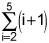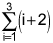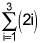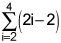2.

Estimate the area under the curve f(x) = x2 from x = 1 to x = 5 by using four inscribed (under the curve) rectangles. Answer to the nearest integer. (4 points)

3.

List x1, x2, x3, x4 where xi is the right endpoint of the four equal intervals used to estimate the area under the curve of f(x) between x = 3 and x = 5. (4 points)

 3, 3.5, 4, 4.5 3, 3.2, 4.6, 5 3.5, 4, 4.5, 5 3.25, 3.75, 4.25, 4.75

4.

Write the summation to estimate the area under the curve y = 2x2 + 1 from x = 0 to x = 4 using 4 rectangles and left endpoints. (4 points)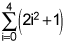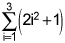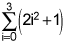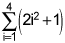5.

If the area under the curve of f(x) = x2 + 2 from x = 1 to x = 6 is estimated using five approximating rectangles and right endpoints, will the estimate be an underestimate or overestimate? (4 points)

 Underestimate Overestimate The area will be exact The area cannot be estimated with just five rectangles

1.

The Riemann sum,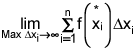, is equivalent to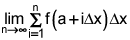with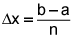.

Write the integral that produces the same value as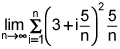. (4 points)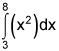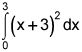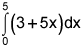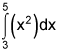2.

Write the Riemann sum to find the area under the graph of the function f(x) = x3 from x = 2 to x = 5. (4 points)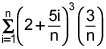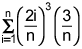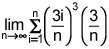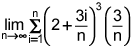3.

Use your calculator to evaluate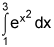. Give your answer to the nearest integer. (4 points)

4.

Use geometry to evaluate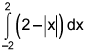. (4 points)

 2 4 8 12

5.

Use geometry to evaluate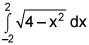. (4 points)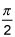π 2π Cannot be evaluated

1.

Given that the antiderivative of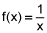is F(x) = Ln|x| + C, evaluate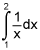. (4 points)

 ln3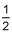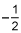ln2

2.

Evaluate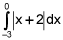. (4 points)

 -2.5 1.5 2.5 3

3.

Given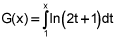, find G '(x). (4 points)

 ln(2x + 1) ln(2x + 1) - ln3 ln(2t + 1) + C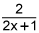4.

Find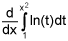. (4 points)

 2xln(x2)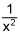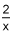ln(x2)

5.

Determine the interval on which f(x) = ln(x) is integrable. (4 points)

 (0, ∞) [0, ∞) (-∞, 0) U (0, ∞) All reals

SkilledTutorZiss
School: UCLAHey, Please find below the full assignment. If you don’t mind going over it quickly, so to make sure everything is right, and we can sign-off on it before your official submission

Which of the following sums does not equal the others? (4 points)

2.
Estimate the area under the curve f(x) = x2 from x = 1 to x = 5 by using four inscribed (under the curve)
rectangles. Answer to the nearest integer. (4 poi...

flag Report DMCAReviewAnonymous
Top quality work from this tutor! I’ll be back!Anonymous
Just what I needed… fantastic!Anonymous
Use Studypool every time I am stuck with an assignment I need guidance.Studypool4.7Trustpilot4.5Sitejabber4.4Brown University

1271 TutorsCalifornia Institute of Technology

2131 TutorsCarnegie Mellon University

982 TutorsColumbia University

1256 TutorsDartmouth University

2113 TutorsEmory University

2279 TutorsHarvard University

599 TutorsMassachusetts Institute of Technology

2319 TutorsNew York University

1645 TutorsNotre Dam University

1911 TutorsOklahoma University

2122 TutorsPennsylvania State University

932 TutorsPrinceton University

1211 TutorsStanford University

983 TutorsUniversity of California

1282 TutorsOxford University

123 TutorsYale University

2325 Tutors